# Angle Sum Property

Angle Sum Property
Go back to  'Triangles'

Max came across a problem of geometry dealing with the angle sum property. He wanted to find the value of $$\angle x$$ in the triangle shown below, when the value of $$\angle BAC=50^{\circ}$$ and $$\angle ABC=90^{\circ}$$.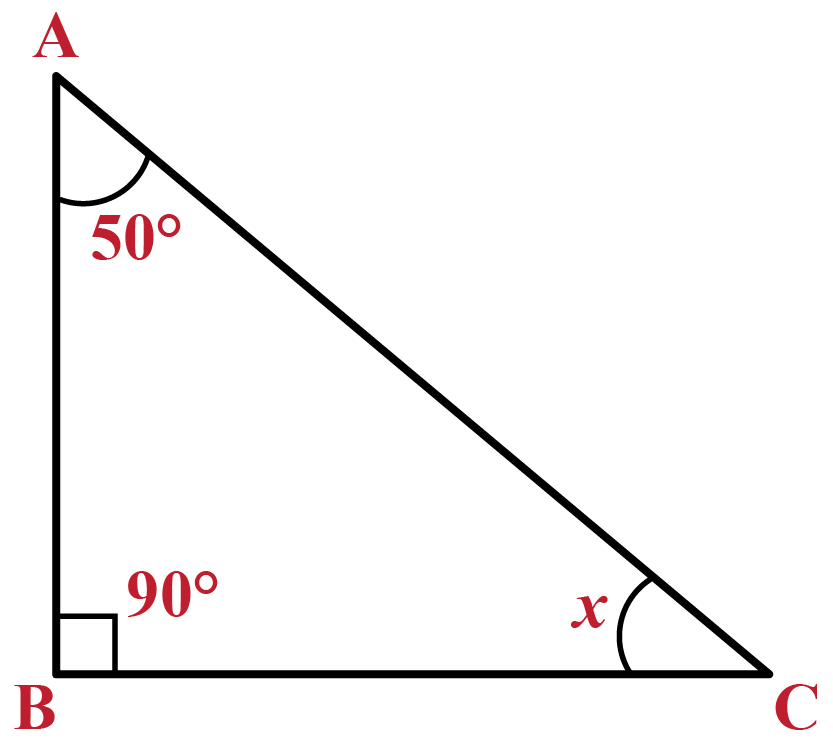His friend, Sam, helped him to solve this by telling him about the angle sum property.

This property states that the sum of all the angles of a triangle is always $$180^{\circ}$$.

In the given $$\triangle ABC$$, $$\angle BAC + \angle CBA + \angle ACB = 180^{\circ}$$

So, $$\angle ACB+90^{\circ}+50^{\circ}=180^{\circ}$$

$$\therefore$$ $$\angle ACB=\angle x=40^{\circ}$$.

## Lesson PLan

 1 What Is Angle Sum Property? 2 Challenging Questions 3 Solved Examples 4 Interactive Questions 5 Important Notes

## What Is Angle Sum Property?

### Definition

The angle sum property states that the sum of the angles of a triangle is equal to $$180^{\circ}$$.

Whether a triangle is an acute, obtuse, or a right triangle, the sum of the angles will always be $$180^{\circ}$$.

This can be represented by the formula:

 In a $$\triangle ABC$$, $$\angle B + \angle A + \angle C = 180^{\circ}$$

This triangle angle sum theorem is useful for finding the measure of an unknown angle when the values of the other two angles are known.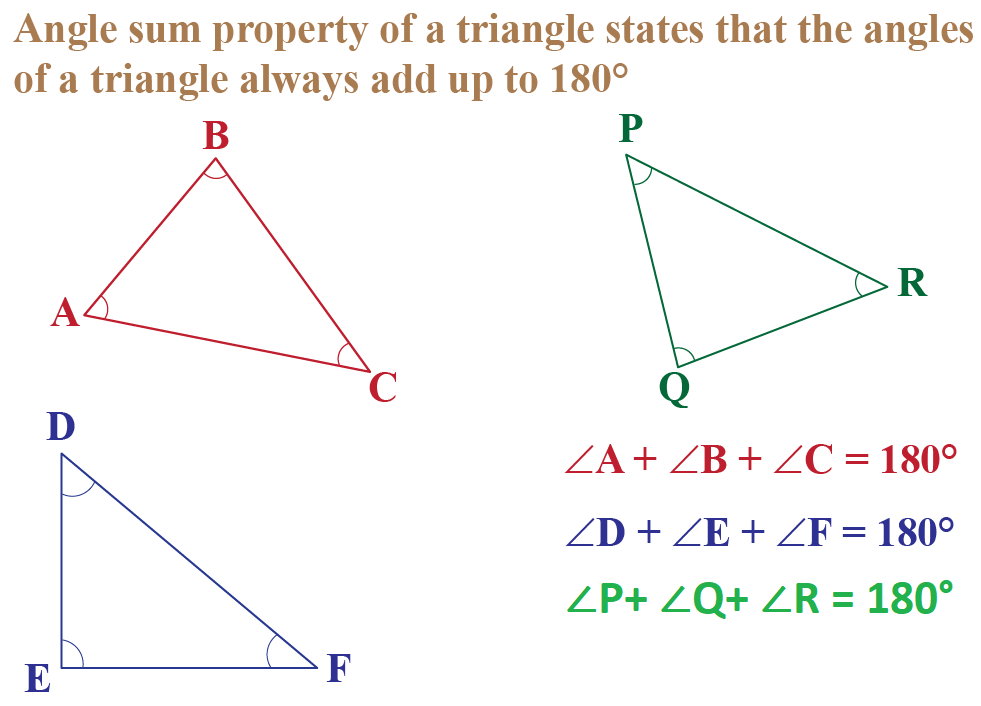## What Is the Exterior Angle of a Triangle?

The exterior angle of a triangle is formed if any side of a triangle is extended.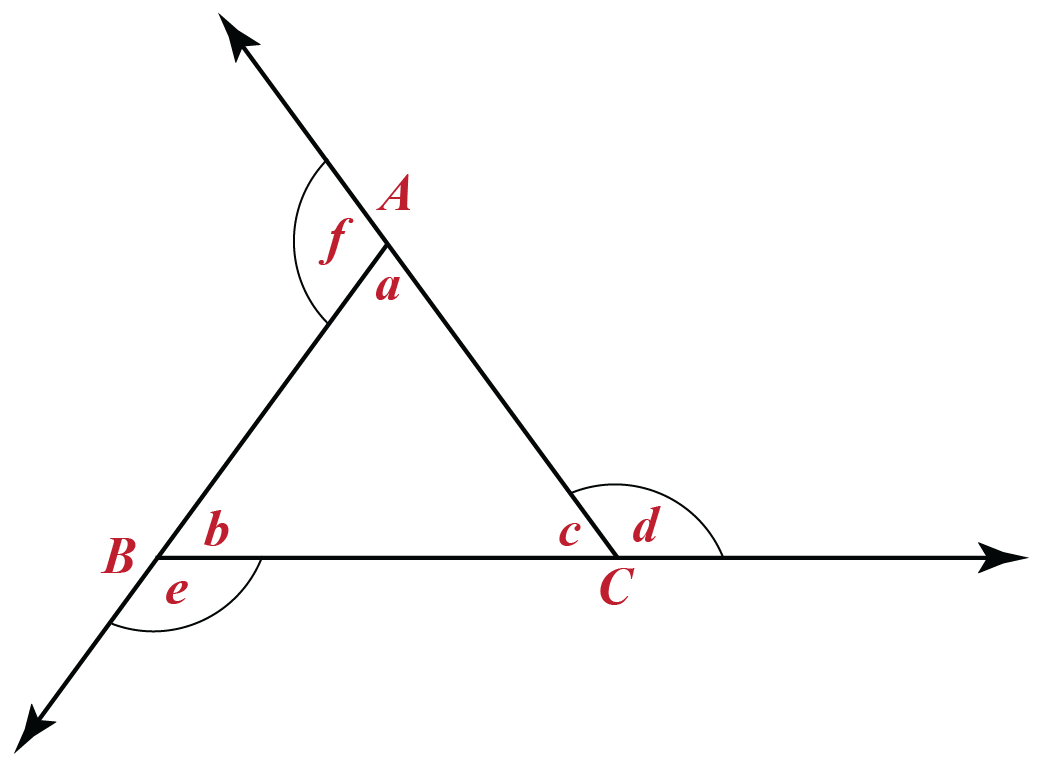In the above image of $$\triangle ABC$$, the interior angles are $$a, b, c$$ and the exterior angles are $$d, e, f$$.

According to the Exterior Angle Property of a triangle, the exterior angle is equal to the sum of the two remote interior angles.

$$i.e.$$, in this case,

$$\angle d = \angle b + \angle a$$

$$\angle e = \angle c + \angle a$$

$$\angle f = \angle b + \angle c$$

## Proof of the Angle Sum Property

### The Steps for Proving the Angle Sum Property of a Triangle

Step 1:
Draw a line $$PQ$$ that passes through the vertex A and is parallel to side $$BC$$ of the $$\triangle ABC$$ as shown below.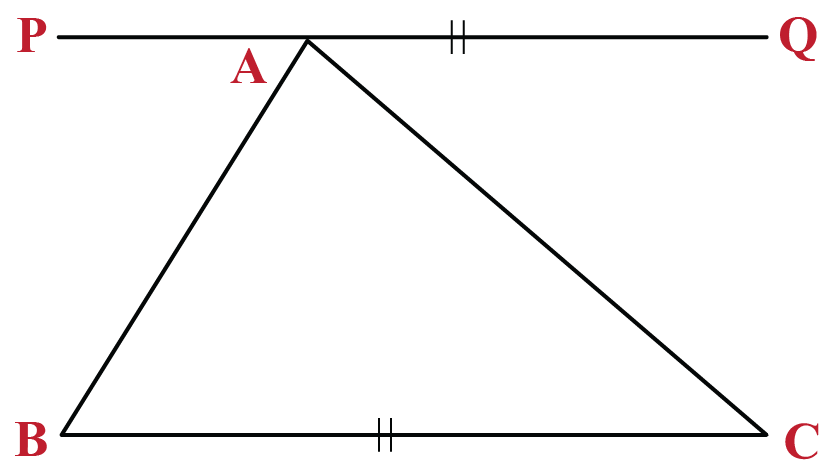Step 2 :
We know that the sum of the angles on a straight line is equal to 180°.

In other words, $$\angle PAB + \angle BAC + \angle CAQ = 180^{\circ}$$

Equation 1: $$\angle PAB + \angle BAC + \angle CAQ = 180^{\circ}$$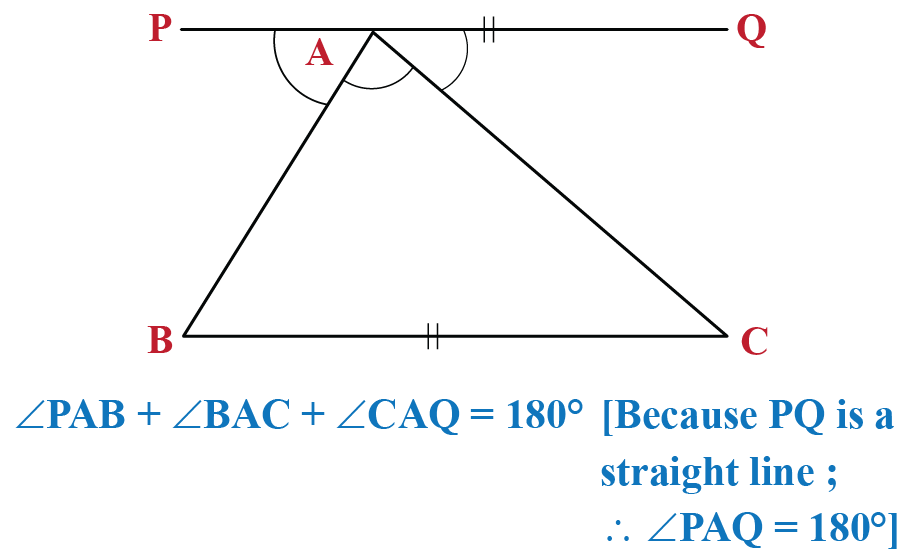Step 3:

Now, since line $$PQ$$ is parallel to $$BC$$

$$\angle PAB$$ = $$\angle ABC$$ and $$\angle CAQ$$ = $$\angle ACB$$.

(Interior alternate angles)

Equation 2: $$\angle PAB$$ = $$\angle ABC$$

Equation 3: $$\angle CAQ$$ = $$\angle ACB$$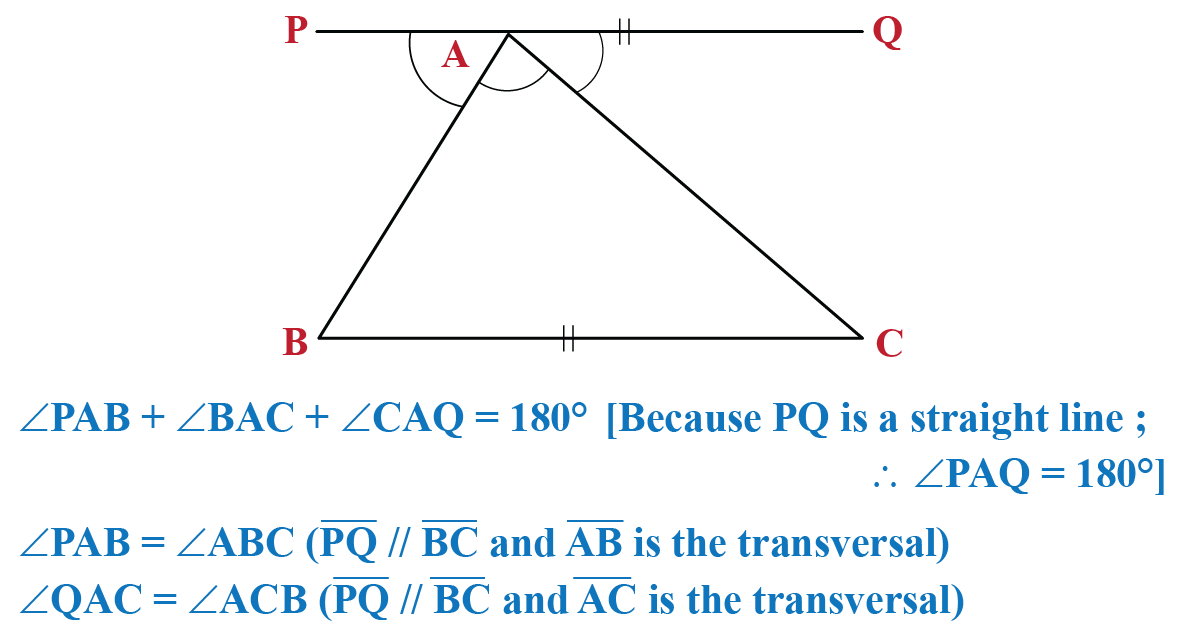Step 4:
Substitute $$\angle PAB$$ and $$\angle CAQ$$ with $$\angle ABC$$ and $$\angle ACB$$ respectively, in Equation 1 as shown below.

Equation 1: $$\angle PAB + \angle BAC + \angle CAQ = 180^{\circ}$$

Thus we get,  $$\angle ABC + \angle BAC + \angle ACB = 180^{\circ}$$

Hence proved, in $$\triangle ABC$$, $$\angle B + \angle A + \angle C = 180^{\circ}$$.

Thus, the sum of all the angles of a triangle is equal to 180°.## Challenging Questions

1. Try to find the angle sum properties of a rectangle, a  hexagon and a pentagon.

## Solved Examples

 Example 1

Sam needs to find the measure of the third angle of a triangle ABC in which $$\angle ABC$$ = 45° and $$\angle ACB$$ = 55°. Can you help him?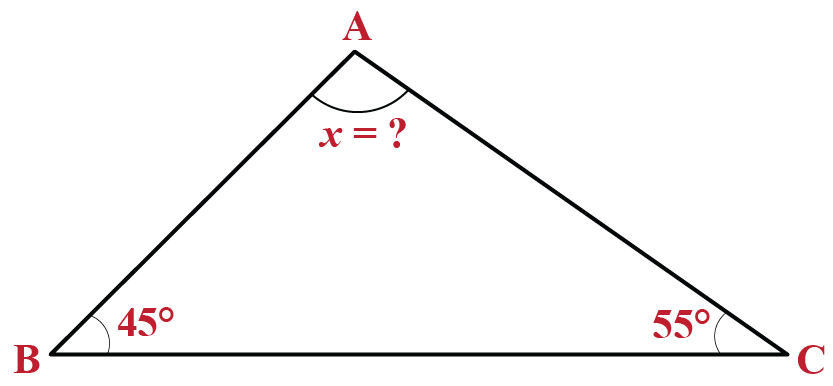Solution

We know that $$\angle ABC =45^{\circ}$$ and $$\angle ACB =55^{\circ}$$

Using the Angle Sum Property of the triangle,

$$\angle A+\angle B+\angle C=180^{\circ}$$.

$$\angle A+45^{\circ}+55^{\circ}=180^{\circ}$$.

$$\angle A+100^{\circ}=180^{\circ}$$.

$$\angle A=180^{\circ}-100^{\circ}$$.

$$\angle A=80^{\circ}$$.

 So, the third angle: $$\angle A=80^{\circ}$$.
 Example 2

If the angles of a triangle are in the ratio $$3:4:5$$, determine the value of the three angles.

Solution

Let the angles be $$3x,\,4x,$$ and $$5x$$

According to the Angle Sum Property of the triangle,

$$3x+4x+5x=180^{\circ}$$.

$$12x=180^{\circ}$$.

$$x=15^{\circ}$$

$$\therefore$$ The three angles will be:

$$3x=3\times 15=45^{\circ}$$

$$4x=4\times 15=60^{\circ}$$

$$5x=5\times 15=75^{\circ}$$

 So, the three angles are $$45^{\circ},\,60^{\circ},\, 75^{\circ}$$.

## Interactive Questions

Here are a few activities for you to practice. Select/Type your answer and click the "Check Answer" button to see the result.

Geometry
grade 9 | Questions Set 1
Geometry
Geometry
grade 9 | Questions Set 2
Geometry